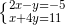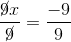Scan QR code or get instant email to install app

Question:

# 1212What is the x value of the solution to the systemA −1
explanation

1212To solve the system by eliminating y, multiply the first equation, 2x – y = –5, by 4 to obtain 8x – 4y = –20. Next, add the second equation, x + 4y = 11, to the transformed equation and then solve the resulting equation for x.
8x − 4y = −20
x + 4y = 11
9x + 0 = −9
9x = −9x = -1